# Diaspora

## Chapter 15: 5+1

### Potential energy wells in 3 and 5 dimensions

In 3-dimensional space (below left), gravitational attraction is inversely proportional to the square of distance. In 5-dimensional space (below right), it’s inversely proportional to the fourth power of distance; the central well is steeper, but further away the surface flattens out more rapidly.

Gravitational potential in 3-dimensional space Gravitational potential in 5-dimensional space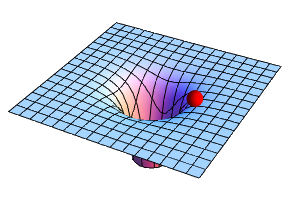Centrifugal force (left) is the same in any number of dimensions: for a given amount of orbital angular momentum, it produces an inverse-cube repulsion.

In 3 dimensions (below left), the combined potential (gravitational plus centrifugal) has an “energy trough” which allows stable circular or elliptical orbits to exist. In 5 dimensions (below right), there is an “energy ridge” instead; this only permits unstable circular orbits, which would need to be maintained artificially. No natural, astronomical object could remain in orbit for long.

Combined potential in 3-dimensional space Combined potential in 5-dimensional space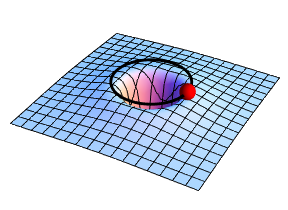### Wave functions in 5 dimensions

In 5 dimensions, the electrostatic attraction between an atom’s positively charged nucleus and its negatively charged lepton (analogous to an electron) follows the same equation as gravity. As a consequence, there are no stable external wave functions, and the lepton wave shrinks to the size of the nucleus. Inside the nucleus, the potential energy well flattens out into a roughly parabolic shape (below left) plus a central peak if the lepton has orbital angular momentum (below right).

Electrostatic potential inside nucleus Combined potential inside nucleus, for lepton with angular momentumThe lowest energy level for the lepton has no orbital angular momentum. The wave function is completely spherically symmetrical, and the probability distribution for any plane slicing the centre of the nucleus is a bell-shaped surface (below left). The next energy level has angular momentum, which drives the wave function away from the centre of the nucleus (below right).

Probability distribution for lowest energy level Probability distribution for next energy level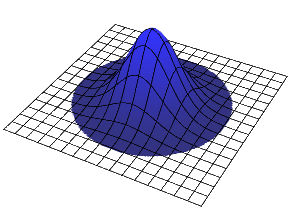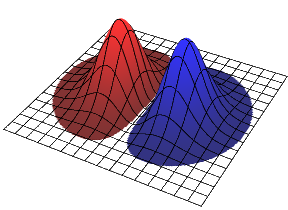The wave function shown above right possesses some angular momentum, but the amount is indefinite. By combining it with the same function rotated 90° (either clockwise or counter-clockwise) and multiplied by the square root of minus one, “eigenfunctions” of angular momentum (wave functions with definite values for that property) can be formed, with values of ±1 unit. These are shown below, with the complex phase of the wave indicated by colour.

 Angular momentum eigenfunctions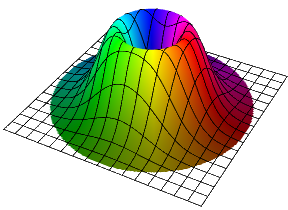Diaspora / Chapter 15: 5+1 / created Saturday, 25 October 1997 / revised Monday, 8 December 1997